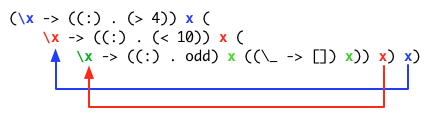# Haskell 'sequence' Over Functions - Explained

Posted by Derek Wyatt on January 25, 2012
A look into how Haskell's Applicative's work. We'll dissect it, along with how it works with the 'sequence' operator.

I’ve recently been devouring Learn You a Haskell for Great Good. I’ve been meaning to do it for ages, but a recent post by Debasish Ghosh pushed me to go through it cover to cover… I’m almost there :) I was wrestling over the complexities of applying the `sequence` function to a list of functions. More succinctly, I was confused on the mechanics of how this worked:

``````ghci> sequence [(> 4), (< 10), odd] 7
[True, True, True]
``````

To figure it out, all you have to do is reduce it, and then reduce it, and then reduce it some more. Let’s do that. First, `sequence` is defined as (I’m using the definition of `sequenceA` from LYAH, as opposed to the definition of sequence from the Haskell API):

``````sequence :: (Applicative f) => [f a] -> f [a]
sequence = foldr (liftA2 (:)) (pure [])
``````

And the definition of the Applicative Functor instance for functions is:

``````instance :: Functor ((->) r) where
pure x = (\_ -> x)
f <*> g = (\x -> f x (g x))
``````

Now, let’s expand (note, I’m not going into nauseating detail here, and assume you’ve gone part of the way thus far):

``````sequence [(> 4), (< 10), odd]
-- becomes
foldr (liftA2 (:)) (pure []) [(> 4), (< 10), odd]
-- which becomes, due to the definition of liftA2
((:) . (> 4)) <*> (((:) . (< 10)) <*> (((:) . odd) <*> (pure [])))
``````

Ok, so we’ve expanded the `foldr` and now we can expand the `<*>` and the `pure []`:

``````(\x -> ((:) . (> 4)) x (
\x -> ((:) . (< 10)) x (
\x -> ((:) . odd) x ((\_ -> []) x)) x) x)
``````

Ok, that’s a bit rough to read… the key to it is noting how the `'x'` gets propagated. Let’s take another look:When we pass in the `7` it gets propagated to all of the little `x`’s, and all of those little `x`’s get passed to their composed functions, and each of those results in a new element on the list:

``````(\x -> ((:) . odd) x ((\_ -> []) x)) 7
-- reduces to
((:) . odd) 7 (\_ -> []) 7)
-- which reduces to
(: True [])
-- which becomes
[True]

-- Now, we use that to reduce the next level up
((:) . (< 10) 7) [True]
-- which becomes
(: True [True])
-- which becomes
[True, True]

-- And now our last level
((:) . (> 4) 7) [True, True]
-- which becomes
(: True [True, True])
-- which becomes
[True, True, True]
``````

Ah, that’s better… now that I ‘get it’, I can move on. Cheers!